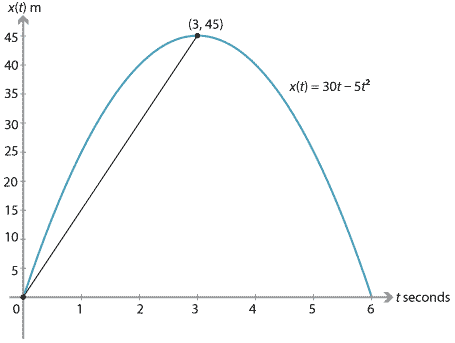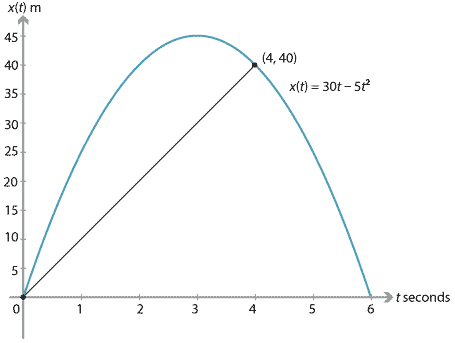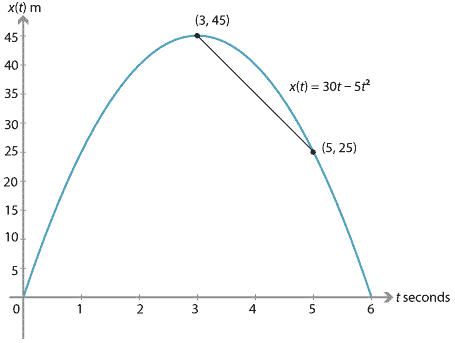## Content

### Average velocity and average speed

Before turning our attention to problems involving non-constant acceleration and using calculus, we consider the concept of average velocity. We will look at examples involving motion with constant acceleration.

#### Average velocity

We return to the example of the stone being launched vertically upwards from ground level with an initial velocity of 30 m/s. The acceleration is taken as $$-10$$ m/s$$^2$$. We found that the stone's velocity is 0 m/s after three seconds, when it is at a height of 45 metres. The average velocity for the first three seconds of motion is

$\dfrac{x(3)-x(0)}{3-0} = \dfrac{45}{3} = 15 \text{ m/s}.$

This can be illustrated on the position–time graph by the chord from the origin to the point $$(3,45)$$. The gradient of this chord is 15.After a further second, the height (position) of the stone is 40 m. That is, $$x(4) = 40$$. The average velocity for the first four seconds is

$\dfrac{x(4)-x(0)}{4-0} = \dfrac{40}{4} = 10 \text{ m/s}.$

This can be illustrated by the chord from the origin to the point $$(4,40)$$. This chord has gradient 10.The average velocity from the time the stone reaches its highest point to when the total time elapsed is 5 seconds is

$\dfrac{x(5)-x(3)}{5-3} = \dfrac{25-45}{2} = -10 \text{ m/s}.$The average velocity from the time the stone is launched to the time it returns to ground level is

$\dfrac{x(6)-x(0)}{6-0} = 0 \text{ m/s}.$

In general, we can write

$\text{Average velocity} = \dfrac{x(t_2)-x(t_1)}{t_2-t_1},$

where $$x(t_i)$$ is the position of the particle at time $$t_i$$.

#### Average speed

The average speed is the distance travelled divided by the time taken:

$\text{Average speed} = \dfrac{\text{Distance travelled}}{\text{Time taken}}.$

Returning to the example of the stone launched upwards with initial velocity 30 m/s:

• the average speed for the first three seconds is $$\dfrac{45}{3} = 15$$ m/s
• the average speed for the first four seconds is $$\dfrac{50}{4} = 12.5$$ m/s
• the average speed from the time the stone reaches its highest point to when the total time elapsed is 5 seconds is $$\dfrac{20}{2} = 10$$ m/s
• the average speed from the time the stone is launched to the time it returns to ground level is $$\dfrac{90}{6} = 15$$ m/s.

Next page - Content - Differential calculus and motion in a straight line